# 数据预处理-数据清理

2020年1月19日 03:22 阅读 1131 评论 0

1. 数据清理：主要是指将数据中缺失的值补充完整、消除噪声数据、识别或删除离群点并解决不一致性。主要是达到如下目标：将数据格式标准化，异常数据清除，错误纠正，重复数据的清除。

2. 数据集成：主要是将多个数据源中的数据进行整合并统一存储。

3. 数据变换：主要是指通过平滑聚集，数据概化，规范化等方式将数据转换成适用于数据挖掘的形式。

4. 数据归约：数据挖掘时往往数据量非常大，因此在少量数据上进行挖掘分析就需要很长的时间，数据归约技术主要是指对数据集进行归约或者简化，不仅保持原数据的完整性，并且数据归约后的结果与归约前结果相同或几乎相同。

## 0x00异常数据分析和处理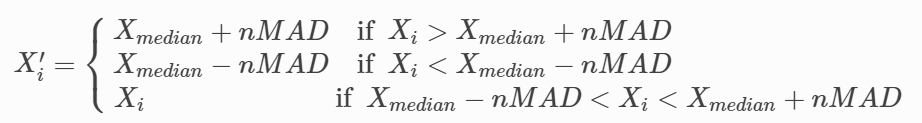- 3σ法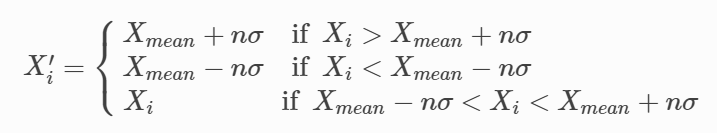- 百分位法：

- 箱型图分析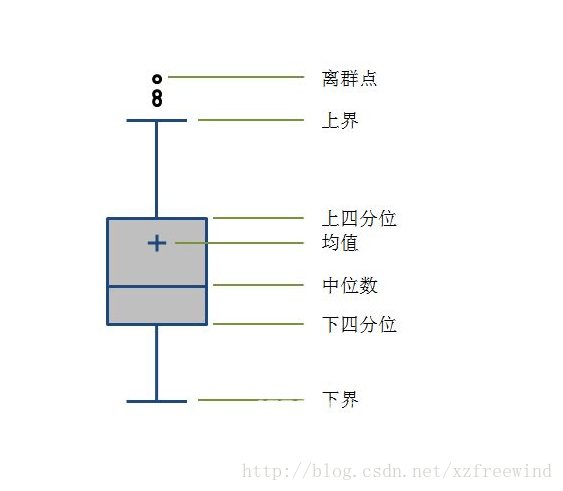1.删除含有异常值的记录

2.将异常值视为缺失值，交给缺失值处理方法来处理

3.用平均值来修正

4.不处理

## 0x01缺失值处理

1.  有些信息暂时无法获取，致使一部分属性值空缺出来。

2.  有些信息因为一些人为因素而丢失了。

3.  有些对象的某个或某些属性是不可用的。如一个未婚者的配偶姓名。

4.  获取这些信息的代价太大，从而未获取数据。

1.  系统丢失了大量的有用信息；

2.  系统的不确定性更加显著，系统中的确定性成分更难把握；

3.  包含空值的数据会使挖掘过程陷入混乱，导致不可靠的输出。

(1)最近邻补插:使用含有缺失值的样本附近的其他样本的数据替代:或者前后教据的平均值替代等。

(2)回归方法:对含有缺失值的属性，使用其他样本该属性的值建立拟合模型， 然后使用该模型预测缺失值。

(3)插值法:和回归法类似，该方法使用已知数据建立合适的插值函数，缺失值使用该函数计算出近似值代替。常见的插值函数有拉格朗日插值法、牛顿插值法、分段插值法、样条插值法、Hermite 插值法等。

## 0x02噪声数据处理

1.噪声是什么？数据集中的干扰数据（对场景描述不准确的数据）

2.噪声怎么产生的？举个例子：手机信号来自于基站发射的电磁波，有的地方比较强，有的地方比较弱。运营商的工程师会负责统计不同区域信号强弱来进行网络规划，工程师采集信号的方法就是将一个信号接受终端固定到车上，然后开车绕着基站转，信号终端就会自动采集不同区域的信号强度，生成一份数据。但是如果车在采集过程中遇到了突发事件、急刹车，就可能会对信号采集造成一定的影响，生成噪声数据。

3.噪声对模型训练有什么影响？很多算法，特别是线性算法，都是通过迭代来获取最优解的，如果数据中含有大量的噪声数据，将会大大的影响数据的收敛速度，甚至对于训练生成模型的准确也会有很大的副作用。

## 0x03去除噪声方法1-正态分布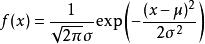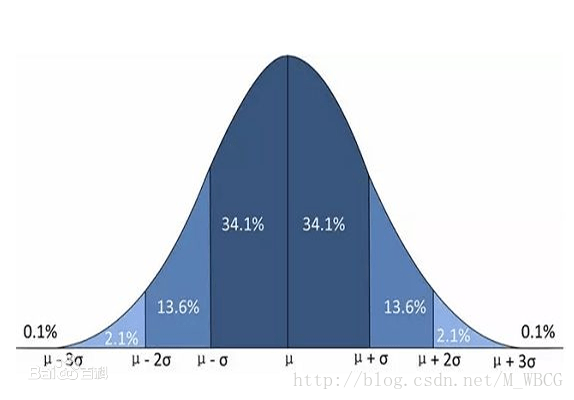```from __future__ import division

mat = [[19, 26, 63], [13, 62, 65], [16, 69, 15], [14, 56, 17], [19, 6, 15], [11, 42, 15], [18, 58, 36], [12, 77, 33],

[10, 75, 47], [15, 54, 70], [10017, 1421077, 4169]]

# 获得矩阵的字段数量

def width(lst):

i = 0;

for j in lst:

i += 1

return i

# 得到每个字段的平均值

def GetAverage(mat):

n = len(mat)

m = width(mat)

num =  * m

for i in range(0, m):

for j in mat:

num[i] += j[i]

num[i] = num[i] / n

return num

# 获得每个字段的标准差

def GetVar(average, mat):

ListMat = []

for i in mat:

ListMat.append(list(map(lambda x: x - x, zip(average, i))))

n = len(ListMat)

m = width(ListMat)

num =  * m

for j in range(0, m):

for i in ListMat:

num[j] += i[j] * i[j]

num[j] /= n

return num

# 获得每个字段的标准差

def GetStandardDeviation(mat):

return list(map(lambda x:x**0.5,mat))

# 对数据集去噪声

def DenoisMat(mat):

average = GetAverage(mat)

variance = GetVar(average, mat)

standardDeviation=GetStandardDeviation(variance)

section = list(map(lambda x: x + 3*x, zip(average, standardDeviation)))

n = len(mat)

m = width(mat)

num =  * m

denoisMat = []

noDenoisMat=[]

for i in mat:

for j in range(0, m):

if i[j] > section[j]:

denoisMat.append(i)

break

if j==(m-1):

noDenoisMat.append(i)

print("去除完噪声的数据：")

print(noDenoisMat)

print("噪声数据：")

return denoisMat

if __name__ == '__main__':

print("初始数据：")

print(mat)

print(DenoisMat(mat))
```

## 0x04去除噪声方法2-分箱

```箱1：800 1000 1200 1500

```

```箱1：800 1000 1200 1500 1500 1800

```

```箱1：800

```

⑴按平均值平滑

⑵按边界值平滑

⑶按中值平滑

## 0x06回归方法

0 人参与 | 0 条评论
暂时没有评论，欢迎来尬聊！